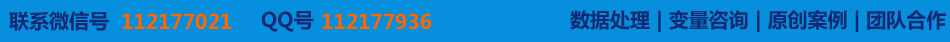# 数学实验算法及MATLAB实现

### 〖此文字数共约:20000以上字文章页数共约:页发布时间:2021年及之前原创指数:〗

【内容概述】

Abstract
The purpose of mathematics experiments course requests a student to set out from the problem , by the help of a computer , experiences the process of solving numerical computation and  the realization of mathematics problems , learns something from the experiment , investigates and discovers the law of mathematics . Mathematics experiments course can raise the interests, consciousnesses and abilities of learning and using mathematics , so as to raise the abilities of  making use of  the  mathematics tools and the computer technique analysis and solving practical problems  , so it has important practical meanings .
The topic researches the basic thought and method of mathematics experiment, and sees mathematics as a course of “experiment science”. We have written experiment algorithm and carried out with MATLAB programs corresponds to each  mathematics problems .At the same time experiment data , pictures and tables are also enclosed herewith to explain the results .
This topic introduces the background and the development of mathematics experiments first; then introduces some simple mathematics experiments and  some synthetic mathematics experiments : simple mathematics experiments introduce  knowledge about differentiation and  integration , the approximation calculating of   , prime numbers and sequences . And synthetic mathematics experiments introduce the problem about heavenly body moving and the problem about personal housing mortgage ; last introduces some MATLAB software knowledge related to the topic.

Keywords　 mathematics experiments ;  numerical  computation ; MATLAB software

Abstract II

1.1 课题背景 1
1.2数学实验课的宗旨和实践意义 1
1.3数学实验与研究性学习的关系 2
1.4数学实验的发展特点 2
1.5数学实验课与其它相关课程的差别与联系 2
1.6本章小结 3

*若需协助请咨询↓→[电脑QQ][手机QQ]【数据协助】